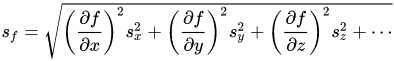# Distance between two uncertain points using haversine?

Frank Einstein
TL;DR Summary
I have two points which represent geographical positions whose coordinates are given in spherical coordinates and also have an associated uncertainty. I can calculate the distance between said points in cartesian coordinates, but I don't know how to translate the uncertainty.
Hello everyone.

I have two points in space (on the surface of the earth) represented using spherical coordinates (in this case there is no z axis since both are assumed to be at the same height). These points have an associated standard deviation in lambda and in phi, which are longitude and latitude respectively. I can calculate the distance between the mean position of both points using the haversine as

ζ=sin^2[(φ1-φ2)/2]+cos(φ1)cos(φ2)sin^2[(λ1-λ2)/2] and then

d=2*R*atan2(√ζ, √1-ζ) where R is the Earth radius and d is the distance in meters between the points defined by (λi,φi) where i=1,2.

However, I have no idea on how to find out the uncertainty of d. I have a standard deviation for the values of (λi,φi), I was wondering if someone can tell me how to incorporate this data to the haversine to be able to calculate the standard deviation of the distance d.

Can someone please tell me of a way to do this?

Any help is appreciated.
Regards.
Frank.

Mentor
2022 Award
What do you mean by uncertainty? If e.g. you have a circle of uncertainty around each point, then uncertainty of distance is plus, minus twice the radius of said circle.

Frank Einstein
What do you mean by uncertainty? If e.g. you have a circle of uncertainty around each point, then uncertainty of distance is plus, minus twice the radius of said circle.
Thanks for the answer. I want to calculate the standard deviation of the variable d knowing the standard deviations of φ1, φ2, λ1 and λ2

Mentor
2022 Award
So you have the random variable ##X=d(\varphi_1,\varphi_2,\lambda_1,\lambda_2 )## where the variables themselves are random variables, and the function is composed of many trigonometric functions. I don't think there is a reasonable closed form for this problem. So you can try to use the Taylor series for the trigonometric functions, which still will leave you with a complicated integral, or you abbreviate this step by the formula given in
https://en.wikipedia.org/wiki/Variance#Approximating_the_variance_of_a_function
or you use one of the formulas in here:
https://en.wikipedia.org/wiki/Distance_correlation

I think it is already difficult to calculate the mean value for the normal distribution function, which I assume is relevant here.

•DaveE and Frank Einstein
Homework Helper
2022 Award
I think it is already difficult to calculate the mean value for the normal distribution function, which I assume is relevant here.

I don't see the difficulty here . This is standard propagation of error for nonlinear system. You just use the partial derivative.

https://en.wikipedia.org/wiki/Propagation_of_uncertainty

Am I missing something?

•DaveE and fresh_42
Frank Einstein
So you have the random variable ##X=d(\varphi_1,\varphi_2,\lambda_1,\lambda_2 )## where the variables themselves are random variables, and the function is composed of many trigonometric functions. I don't think there is a reasonable closed form for this problem. So you can try to use the Taylor series for the trigonometric functions, which still will leave you with a complicated integral, or you abbreviate this step by the formula given in
https://en.wikipedia.org/wiki/Variance#Approximating_the_variance_of_a_function
or you use one of the formulas in here:
https://en.wikipedia.org/wiki/Distance_correlation

I think it is already difficult to calculate the mean value for the normal distribution function, which I assume is relevant here.
Thanks again for the answer. That's what I feared, no closed loop form. I don't know if this is relevant, but I am working in Matlab, where I have calculated the aforementioned quantities as

Distance calculation:
haversine_angle=(sin((phiMeanA-phiMeanB)/2)).^2+cos(lammdaMeanA).*cos(lammdaMeanB).*(sin((lammdaMeanA-lammdaMeanB)/2)).^2;
haversine_sqrt=(haversine_angle).^0.5;
haversine_sqrt2=1.-haversine_sqrt;

haversine_mean_distance =RT.*atan2(haversine_sqrt, haversine_sqrt2);
and the standard deviations are in different variables. The values of the standard deviations are

phiStdA=6.608673643811970e-08

phiStdB=7.548195779015499e-08

lambdaStdA= 7.757704741906700e-09

lambdaStdB=9.494186140546169e-09

and the values of the position of the mean of both points is

phiMeanA=0.879103498447014

phiMeanB=0.856643114991405

lammdaMeanA=0.007900824598177

lammdaMeanB=0.007461768949733

haversine_mean_distance=7.244901394376239e+04

RT=6378000

Frank Einstein
I don't see the difficulty here . This is standard propagation of error for nonlinear system. You just use the partial derivative.

https://en.wikipedia.org/wiki/Propagation_of_uncertainty

Am I missing something?
Probably I am the one missing something here. I don't know how to implement what you are providing since I only have numerical values as you can see in my previous message

Frank Einstein
Yes, but there is nothing in said page which explains how to find the standard deviation ong the distance d having the uncertainty of both points expressed in spherical coordiantes.

Homework Helper
2022 Award•Frank Einstein
Frank Einstein

View attachment 277152
So, if I understand you correctly, I have to manually derivate atan2(√ζ, √1-ζ) respect to φ and then respect to λ and then substitute the values in the point as
phiStdA=6.608673643811970e-08

phiStdB=7.548195779015499e-08

lambdaStdA= 7.757704741906700e-09

lambdaStdB=9.494186140546169e-09

and the values of the position of the mean of both points is

phiMeanA=0.879103498447014

phiMeanB=0.856643114991405

lammdaMeanA=0.007900824598177

lammdaMeanB=0.007461768949733

Is this it? I thought that I could not calculate that with at least two points to have a value of the derivative. I don't know what I was thinking.

Thanks.
Regards.
Frank.

Please, confirm me that I have the right idea.

•